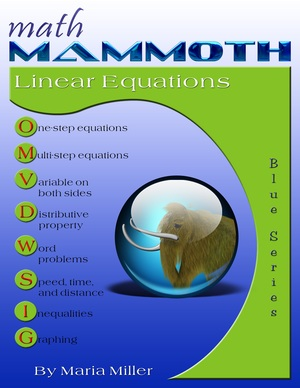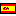^
You are here: HomeBlue Series → Linear Equations

# Math Mammoth Linear Equations140 pages

## Prices & ordering

Printed copy \$17.65

(credit cards, Google Pay, Paypal, PO)Este libro en español

Math Mammoth Linear Equations presents the student with the basics of solving linear equations, including equations that involve a variable on both sides and equations that require the usage of the distributive property to eliminate parentheses. We also briefly study inequalities and graphing. This book is best suited for pre-algebra or grades 7-8 mathematics studies.

The first lesson reviews the concept of an equation and how to model equations using a pan balance (scale). The basic principle for solving equations is that, when you perform the same operation on both sides of an equation, the two sides remain equal.

The book presents two alternatives for keeping track of the operations to be performed on an equation. The one method, writing the operation under each side of the equation, is common in the United States. The other method, writing the operation in the right margin, is common in Europe. Either way is correct, and the choice is just a matter of the personal preference of the teacher.

The introduction to solving equations is followed by a lesson on addition and subtraction equations and another on multiplication and division equations. All the equations are easily solved in only one step of calculations. The twofold goal is to make the student proficient in manipulating negative integers and also to lay a foundation for handling more involved equations that are studied later on in the book.

In the next lesson, students write equations to solve simple word problems. Even though they could solve most of these problems without using the equations, the purpose of the lesson is to make the student proficient in writing simple equations before moving on to more complex equations from more difficult word problems.

The next topic, in the lesson Constant Speed, is solving problems with distance (d), rate or velocity (v), and time (t). Students use the equivalent formulas d = vt and v = d/t to solve problems involving constant or average speed. They learn an easy way to remember the formula v = d/t from the unit for speed that they already know, "miles per hour".

In later lessons, we delve deeper into our study of equations. Now the equations require two or more steps to solve and may contain parentheses. The variable may appear on both sides of the equation. Students will also write equations to solve simple word problems.

There is also a lesson on growing patterns, which may simply seem to be a fascinating topic, but in reality presents the fundamentals of a very important concept in algebra – that of linear functions (although they are not mentioned by that name) – and complements the study of lines in the subsequent lessons.

After the section about equations, the text briefly presents the basics of inequalities and how to graph them on a number line. Students apply the principles for solving equations to solve simple inequalities and word problems that involve inequalities.

The last major topic is graphing. Students begin the section by learning to graph linear equations and continue on to the concept of slope, which in informal terms is a measure of the inclination of a line. More formally, slope can be defined as the ratio of the change in y-values to the change in x-values. The final lesson applies graphing to the previously-studied concepts of speed, time, and distance through graphs of the equation d = vt in the coordinate plane.

## Better Yet - Bundle Deals!

*BONUS*: Buy any bundle listed above, and get the fully upgraded Soft-Pak software for FREE (includes 4 math and 2 language arts programs, complete with on screen and printable options).

## How and where to order

You can buy Math Mammoth books at:

• Here at MathMammoth.com website — simply use the "Add to cart" buttons you see on the product pages.
• Rainbow Resource carries printed copies for the Light Blue series books, plus several CDs (Light Blue and Blue series).
• At Teachers Pay Teachers you can purchase the Light Blue Series downloads, plus topical units.
• Lulu sells printed copies for most of the Math Mammoth materials (various series).

By purchasing any of the books, permission IS granted for the teacher (or parent) to reproduce this material to be used with his/her students in a teaching situation; not for commercial resale. However, you are not permitted to share the material with another teacher.

In other words, you are permitted to make copies for the students/children you are teaching, but not for other teachers' usage.

Math Mammoth books are PDF files. You will need Adobe Reader to view them, including if you use a Mac or Linux. You can try other PDF viewers, but they seem to either omit or mess up some of the images.
WAIT!

Receive my monthly collection of math tips & resources directly in your inbox — and get a FREE Math Mammoth book!You can unsubscribe at any time.

### Math Mammoth Tour

Confused about the different options? Take a virtual email tour around Math Mammoth! You'll receive:

An initial email to download your GIFT of over 400 free worksheets and sample pages from my books. Six other "TOURSTOP" emails that explain the important things and commonly asked questions concerning Math Mammoth curriculum. (Find out the differences between all these different-colored series!)

This way, you'll have time to digest the information over one or two weeks, plus an opportunity to ask me personally about the curriculum.
A monthly collection of math teaching tips & Math Mammoth updates (unsubscribe any time)### "Mini" Math Teaching Course

This is a little "virtual" 2-week course, where you will receive emails on important topics on teaching math, including:

- How to help a student who is behind
- Troubles with word problems
- Teaching multiplication tables
- Why fractions are so difficult
- The value of mistakes
- Should you use timed tests
- And more!

A GIFT of over 400 free worksheets and sample pages from my books right in the very beginning.A monthly collection of math teaching tips & Math Mammoth updates (unsubscribe any time)
Enter your email to receive math teaching tips, resources, Math Mammoth news & sales, humor, and more! I tend to send out these tips about once monthly, near the beginning of the month, but occasionally you may hear from me twice per month (and sometimes less often).• A GIFT of over 400 free worksheets and sample pages from my books.